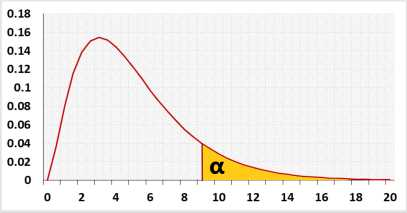# Kaplan Meier Survival Analysis

## Kaplan Meier online and log rank test calculator

Generates the Kaplan-Meier plot and calculates the log-rank test.

Please ensure every participant has an event or censored event by the end of the study.

Enter raw data directly
Enter raw data from excel
Enter aggregated data
 Time Events Censors Group

The tool will trim empty cells, spaces or non-numeric data in the numeric columns.
If you enter only one group the log-rank test will not run.
You may use more than two groups.
To download a plot as png, press the camera icon on any Kaplan Meier curve.

## Information

The Kaplan–Meier method is a non parametric method used for the survival analysis. The survival probability calculator generates the Kaplan-Meier curve with confidence interval and calculates the Log-Rank test for more than of two groups.
Event of interest (Dt): Maintenance failure, recovery, disease occurrence, death, etc.
Censored event (Ct): the event of interest didn't happen since the subject left the experiment before the ending time, or due to the termination of the experiment.
nt: number of participants that didn't have an event yet (event of interest or censored event).
Proportion surviving interval (Pt): the proportion of survival participants between period t-1 and period t.
Survival rate (St / Cumulative Survival/ Survival function): the proportion of survival participants from period 0 to period t.
Sum = ΣDt/nt(nt - Dt)
S.Et: the standard deviation of the survival rate. S.E = St√(Sum)
Lowert: Lower bound of St confidence interval .
Uppert: Upper bound of St confidence interval.

### Chart type

Line –Survival rate (St) per time.
Confidence interval – added the Confidence interval areas to the line, with confidence level of (1 - α).
S.E area – present St ± 1*S.E, the S.E is the area below and above the line.
S.E error bars – present St ± 1*S.E, the S.E is presented as error bar.

### Log rank test calculator

Target: test the null assumption that the "time to event" distribution is identical for all the groups.
The test uses the chi-square distribution.

The log-rank test model assumes the events per subject distributes evenly between the groups. The expected number of events is calculated per each time value.
Example with two groups A and B.
Expected value = nA(dA + dB)/(nA + nB)

The page was created per Anna P request.
Hypotheses
H0: Equal survival distributions
H1: Unequal survival distribution
Test statisticχ² distribution